# ChIPS - displaying image dataChIPS Threads (CIAO 4.11 ChIPS v1)

## Overview

#### Synopsis:

This thread is intended to give a simple demonstration of the support for displaying image data in ChIPS.

Last Update: 15 Dec 2015 - Updated for CIAO 4.8.

## Introduction

The Starting ChIPS thread describes how to start ChIPS. Please see the ChIPS GUI section of that thread for information on the ChIPS GUID.

The data used in this thread is available in the chips_data.tar.gz file.

```unix% ls -1 chips/image/
a4059_chandra.fits@
a4059_chandra_bin1.fits@
a4059_fov1.fits@
a4059_galex.fits@
```

The data used in this thread is similar to the Introduction to Contours thread.

## Displaying an image

Here we use the make_figure command to display the image data for the file a4059_chandra_bin1.fits. We need to explicitly give the type of plot (i.e. "image") as an argument otherwise the data would have been displayed as a contour.

```chips> make_figure("a4059_chandra_bin1.fits", "image")
chips> print(info())
Window [win1]
Frame [frm1]
Plot [plot1]   (0.15,0.15)  .. (0.90,0.90)
Border bottom [bx1]  top [bx2]  left [by1]  right [by2]
Image [image1]
X Axis [ax1]
Y Axis [ay1]

chips> print(get_image())
alpha = [1.0, 1.0]
colormap = 3
colormap_interpolate = True
colormap_size = 256
depth = 100
id = None
interpolation = 0
invert_colormap = False
scale_channels = True
stem = None
threshold = [0.0, 37.0]

```

The result is shown in Figure 1. When axes are created to display an image, the axis pad attribute is ignored. This differs from displaying curves, histograms and contours, which will use the default pad value of axes.

### Figure 1: Basic display of a4059_chandra_bin1.fits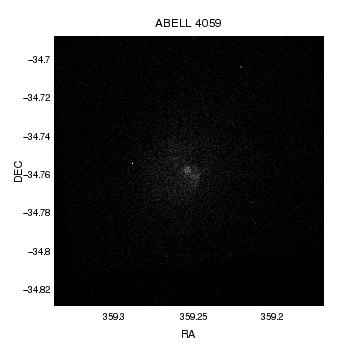### Figure 1: Basic display of a4059_chandra_bin1.fits

The make_figure command has created an image of the data in a4059_chandra_bin1.fits. The default is to use the grayscale color map and to display the full data range of the image; we show below how to change both these options. We also show how to stop the axes from being hidden by the image, and issues related to the hardcopy (e.g. PS and PNG) output.

By default, objects are drawn at a depth of 100, but we can change the value - and hence the order they are displayed - using the relevant set_<object> call. Here we move the image behind the axes (objects with a larger depth are drawn after lower depth items).

```chips> set_image(["depth", 50])
```

Note that we can also change the order items are draw within a depth level using the various shuffle_<object> routines; for instance using

`chips> shuffle_image(chips_back)`

You can also shuffle an object by selecting it with the mouse and then using the Shuffle Object item from the right-mouse-button menu in the ChIPS window.

The depth of the objects can be found using the info_depth command, as shown below:

```chips> print(info_depth())
Window [win1]
Frame [frm1]
Depth 50
image [image1 (plot1)]
Depth 100
label [title (plot1)]
axis [bx1 (plot1)]
axis [bx2 (plot1)]
axis [by1 (plot1)]
axis [by2 (plot1)]
axis [ax1 (plot1)]
axis [ay1 (plot1)]

```

We now change the colormap used to display the image:

```chips> set_image(["colormap", "heat"])
```

ChIPS includes several inbuilt color maps and the ability to load in user-defined versions. The set_image call will return an error if an invalid color map name is used, as shown below:

```chips> set_image(["colormap", "a"])
chips ERROR: The colormap value (a) must be one of [red, green, blue, grayscale, rainbow, hsv, heat, cool, usercmap1, usercmap2, usercmap3]
```

Finally we change the display range of the data. In CIAO 4.8 ChIPS only supports a linear display range (in later examples we show how this limitation can be worked around), and we use the threshold attribute to specify the minimum and maximum range to display. We first use the dmstat tool to find the range of the data (a line starting with ! runs the rest of the line as a shell command from ChIPS and Sherpa):

```chips> !dmstat a4059_chandra_bin1.fits cen-
IMAGE
min:        0             @:        ( 3557.64 3735.09 )
max:        37            @:        ( 4304.64 4127.09 )
mean:        0.6431097561
sigma:        1.0559878309
sum:        675008
good:        1049600
null:        0
chips> set_image(["threshold", [0,15]])
```

which results in Figure 2.

The ChIPS GUI makes it easy to manipulate your visualization; for this visualization the GUI looks like Figure 3.

### Figure 2: Changing the color map and threshold of the image### Figure 2: Changing the color map and threshold of the image

Changing the color map and threshold of the image has made the data more visible. The axes (e.g. the tick marks) are now visible in the on-screen version of the image (compare to Figure 1) but they are not in the hardcopy versions (they are drawn using the default color, which means black in the PNG, PS, or PDF versions, which is hard to see against the black of the image).

The coordinates of the mouse pointer are always displayed in the ChIPS window title when the window is active, as long as this is supported by the window manager.

### Figure 3: The ChIPS GUI### Figure 3: The ChIPS GUI

The ChIPS GUI can be displayed by either entering the command

`chips> show_gui()`

at the prompt or by using the Show GUI option from the right-mouse-button menu in the ChIPS window.

See ahelp chipsgui for more information.

One way to make the tick marks visible is to move them to point out of the plot, rather than into it. We do this for all the axes, which ensures that the borders also change (that is the top and right axes in this plot). The result of the following command is shown in Figure 4.

```chips> set_axis("all", ["majortick.style", "outside", "minortick.style", "outside"])
```

### Figure 4: Moving the tick marks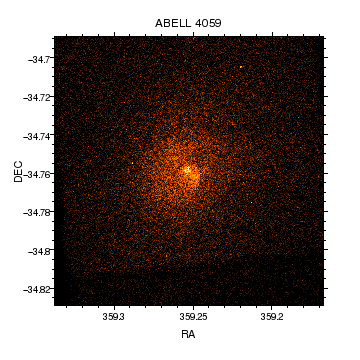### Figure 4: Moving the tick marks

The tick marks are now visible in the hardcopy versions of the plot. An alternative approach would have been to change their color - e.g. using:

```set_axis("all", ["majortick.color", "white", "minortick.color", "white"])
```

Since the image is displayed using the WCS information in the file, it is displayed with a fixed aspect ratio; the limits shown in Figure 4 are chosen so as to retain this ratio. Note that the plot aspect ratio is unset, which means that the limits will always be chosen so that the pixels remain square but that the plot can be resized to any shape.

```chips> get_data_aspect_ratio()
'1:1'
chips> get_plot_aspect_ratio()
''
```

The affect of the aspect ratio is seen below, where the Y limits are automatically changed when the X limits are adjusted. This can also be seen when using pick_limits to interactively select the region to display, where the dotted rectangle displays the region that matches the aspect ratio of the data. Further discussion of aspect ratios is left to the coordinates-systems section.

```chips> get_plot_yrange()
[-34.829038613354008, -34.68896175153688]
chips> limits(X_AXIS, 359.3, 359.2)
chips> get_plot_xrange()
[359.29999999999518, 359.19999999999612]
chips> get_plot_yrange()
[-34.800076032076817, -34.717924293060499]
```

Rather than using the limits call above, we decide to use panto to re-center the image and the zoom to change the plot limits. We could have also taken advantage of the interactive capabilities of the ChIPS GUI to pan and zoom directly using the mouse.

```chips> undo()
chips> panto(359.255, -34.76)
chips> zoom(1.5)
chips> get_plot_xrange()
[359.311873188108, 359.1981911210496]
chips> get_plot_yrange()
[-34.806678049748193, -34.713295551808372]
```

We now customize the plot to improve the labeling of the axes. The resulting figure is shown in Figure 5:

```chips> set_xaxis(["tickformat", "ra"])
chips> set_yaxis(["tickformat", "dec"])
```

### Figure 5: Adjusting the plot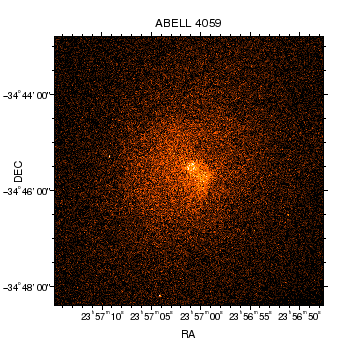### Figure 5: Adjusting the plot

There are a variety of formats for displaying axes, including several sexagesimal ones. The ra and dec formats mean that the numeric labels on the axes are displayed as 23h57m10s and -34°48'00".

The coordinates displayed in the ChIPS window title are displayed using the axis format - compare to Figure 4 - although note that all sexagesimal formats use the colon-separated form in the window title.

If we use the ra2 and dec2 formats we change the axis labeling to the form h m s and d m s (i.e. spaces as separators for the sexagesimal components).

```chips> set_xaxis(["tickformat", "ra2"])
chips> set_yaxis(["tickformat", "dec2"])
```

As annotation, we add a label to the bottom-left corner of the plot. By setting the coordsys attribute we explicitly select the coordinate system for the label (if not given it would have used the current coordinate system, which is the WCS axes of the image). The PLOT_NORM system is a linear system where the bottom-left corner of the plot is (0,0) and the top-right corner is (1,1). The resulting plot is shown in Figure 6.

```chips> add_label(0.1, 0.1, "Chandra", ["coordsys", PLOT_NORM, "size", 18])
chips> print(info_coordinate())
Window [win1]
Frame [frm1]
Plot [plot1]
Coord Sys ID [ds0.0.0.3]
X Axis [ax1]
Y Axis [ay1]

```

### Figure 6: Adding a label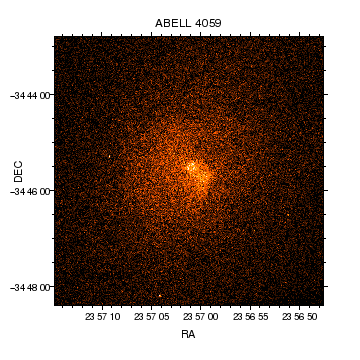### Figure 6: Adding a label

The label is positioned in the bottom left of the plot using the plot-normalized coordinate system. As with the axis tick labels in Figure 2, the label uses the default color which is very hard to see in the hardcopy versions (e.g. PDF).

The convert_coordinate command can be used to convert between different coordinate systems: so the plot-normalized coordinate of 0.1,0.1 is, in the current data coordinate system

```chips> convert_coordinate([0.1, 0.1], PLOT_NORM)
[ 359.3004934   -34.79734456]
```

You can also add annotations - such as labels and lines - directly from the GUI.

To make sure that the label is visible in the hardcopy formats we set its color to white:

```chips> set_label(["color", "white"])
```

The pick and get_pick routines allow you to select points on a plot and returns their coordinates; pick prints the values to the screen while get_pick returns python values about the selected points. In the following we show both commands, selecting different points in the image (as we call the routines with no arguments they only respond to one event and then return):

```chips> pick()
(23:57:09, -34:45:19)
chips> coords = get_pick()
chips> len(coords)
5
chips> coords
array([ 359.26755655])
chips> coords
array([-34.80378767])
chips> coords
array(, dtype=uint32)
chips> print(coords)
['23:57:04']
chips> print(coords)
['-34:48:14']
```

The pick and get_pick() display coordinate values in the format used for the axis tickformat attribute (for sexagesimal values they use the format a:b:c for any of the ra or dec options). Since get_pick returns a set of values, the formatted values are the last two elements (coords and coords above).

The coordinates of the mouse are always displayed in the ChIPS window title (if displayed by your window manager).

We now add another annotation to the image; this time a circle (which we approximate using a 50-sided region). The default fill color of green is changed to white, which creates Figure 7.

```chips> x0 = 359.24531689
chips> y0 = -34.75232151
chips> r = 0.006
chips> add_region(50, x0, y0, r)
chips> set_region(["fill.color", "white"])
chips> get_region()

angle = 0.0
depth = 100
edge.color = green
edge.style = 1
edge.thickness = 1.0
fill.color = white
fill.style = 1
id = None
opacity = 0.5
stem = None

```

### Figure 7: Adding a region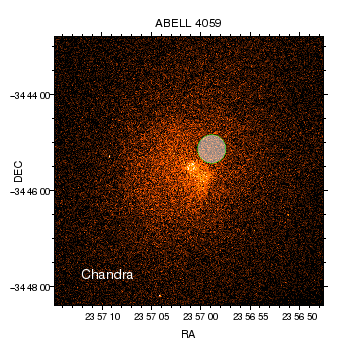### Figure 7: Adding a region

In the screen, PDF, and bitmap versions of the visualization the region fill is transparent (the default opacity is 0.5 as shown in the get_region call above), but it is solid in the postcript version.

Since the label color was changed to white it is now visible in the hardcopy formats (e.g. PNG).

We remove the fill style for the region by changing the fill.style attribute to nofill:

```chips> set_region(["fill.style", "nofill", "edge.color", "white", "edge.thickness", 2])
```

By selecting the region with the mouse, you can bring up a window to edit its properties using the Edit Region item of the right-mouse-button menu, as shown in Figure 8.

### Figure 8: The ChIPS GUI: attribute window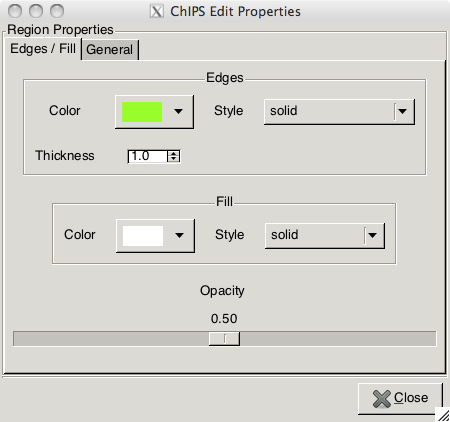### Figure 8: The ChIPS GUI: attribute window

Here we have used the right-mouse-button menu in the ChIPS window to select the Edit Region menu item.

As with image colormaps, an invalid fill style will cause an error listing the valid inputs:

```chips> set_region(["fill.style", "a"])
chips ERROR: The fill style value (a) must be one of [nofill, solidfill, updiagonal, downdiagonal, horizontal, vertical, crisscross, brick, grid, hexagon, polkadot, flower, userfill1, userfill2, userfill3]
```

To complete the plot we first re-center on the cluster (actually, the region we just added) and zoom in:

```chips> panto(x0, y0)
chips> zoom(2)
```

which creates Figure 9.

### Figure 9: Zooming in on the region### Figure 9: Zooming in on the region

Since the label was positioned in plot-normalized coordinates, it remains in the same position relative to the plot no matter how the data limits are changed. If the label had been positioned in data coordinates - e.g. at 359.3004934,-34.79734456 - then it would not be visible in this version of the plot.

The new data coordinates for the label are:

```chips> convert_coordinate([0.1, 0.1], PLOT_NORM)
[ 359.26805414  -34.77099771]
```

Then we move the label to the top of the plot, to create the final plot (Figure 10) (note that here we move to an absolute location and that objects can only be moved using the coordinate system that they were created in). We then decide to add a colorbar to the top of the plot, so we remove the plot title and change the text displayed by the label:

```chips> move_label(0.1, 0.9)
chips> set_label_text("Abell 4059")
chips> set_plot_title("")
chips> add_colorbar(0.5, 1.05)
chips> set_colorbar(["length", 0.8])
```

We also decide to adjust the y-axis label so that it does not overlap the central ticklabel value, and create full-page postscript and PDF formats of the visualization using:

```chips> set_yaxis(["y.label.angle", 0, "label.valign", 1])
chips> print_window("makefig.pdf", ["fittopage", True])
chips> print_window("makefig.ps", ["fittopage", True])
```

### Figure 10: Adding a color bar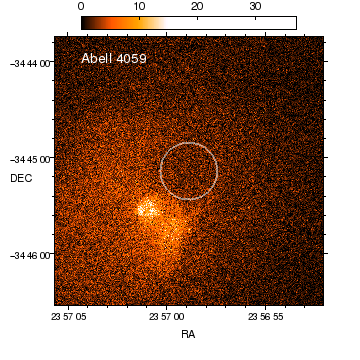### Figure 10: Adding a color bar

The plot title has been removed, with the contents moved to the label, so that a color bar can be added above the plot. The coordinate system used for add_colorbar is the plot-normalized one, so the bar is centered horizontally and just above the plot. The length of the plot is then reduced from its default value of 1 to 0.8. Note that color bars can also be placed vertically, by setting or changing their orientation attribute, as shown below (Figure 23).

## Coordinate systems, axis grids and aspect ratios

In this section we explore the support for displaying different coordinate systems and the use of the plot and data aspect ratios.

### Displaying grid lines

We start by displaying the image and turning on the majorgrid lines, with the result being shown in Figure 11:

```chips> erase()
chips> f = "a4059_chandra_bin1.fits"
chips> add_image(f, ["depth", 50])
chips> set_image(["colormap", "cool", "threshold", [0,15]])
chips> set_image(["invert_colormap", True])
chips> set_axis(["majorgrid.visible", True, "majorgrid.color", "orange"])
chips> zoom(0.5)
```

### Figure 11: Displaying grid lines on an image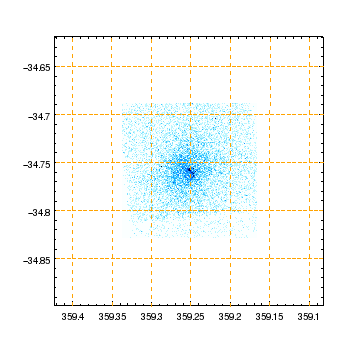### Figure 11: Displaying grid lines on an image

The orange dashed lines indicate lines of constant Right Ascension or Declination.

Tangent-plane WCS projections create their own type of axes to support the non-cartesian nature of the projection. This is not strongly obvious in this figure, since the distortations at this declination are not strong, but it is more obvious in other examples or if you try zooming out further.

The major tick positions change with the tick format, as shown in Figure 12, which was created by saying

```chips> set_xaxis(["tickformat", "ra1"])
chips> set_yaxis(["tickformat", "dec1"])
```

### Figure 12: Changing the tick format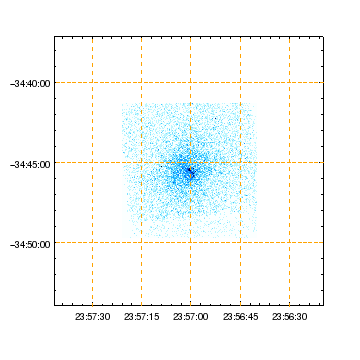### Figure 12: Changing the tick format

With the change to sexagesimal notation (the ra1/dec1 formats use a : to separate the componentns) the grid lines change position compared to those in Figure 11.

In CIAO 4.8, WCS axes do not support all the majortick modes that normal axes do. As an example, the following call fails:

```chips> set_xaxis(["majortick.interval", 0.1])
chips ERROR: WCS axes do not support the axis mode interval. They currently only support limits and nice modes.
```

### Coordinate systems

Now we try displaying the same image as above but this time using the logical and SKY coordinate systems rather than the EQPOS system. The coordinate systems are shown in the dmlist output below:

```chips> !dmlist a4059_chandra_bin1.fits cols

--------------------------------------------------------------------------------
Columns for Image Block IMAGE
--------------------------------------------------------------------------------

ColNo  Name                 Unit        Type             Range
1   IMAGE[1025,1024]                  Int4(1025x1024) -

--------------------------------------------------------------------------------
Physical Axis Transforms for Image Block IMAGE
--------------------------------------------------------------------------------

Group# Axis#
1   1,2    sky(x) = (+3557.140) +(+1.0)* ((#1)-(+0.50))
(y)   (+3734.590)  (+1.0)  ((#2) (+0.50))

--------------------------------------------------------------------------------
World Coordinate Axis Transforms for Image Block IMAGE
--------------------------------------------------------------------------------

Group# Axis#
1   1,2    EQPOS(RA ) = (+359.2482)[deg] +TAN[(-0.000136667)* (sky(x)-(+4096.50))]
(DEC)   (-34.7795 )           (+0.000136667)  (   (y) (+4096.50))
```

The choice of coordinate system is made by changing the wcs attribute. First we try the logical system which uses the FITS pixel coordinates where the bottom left pixel is centered at (1,1), as shown in Figure 13.

```chips> erase()
chips> add_image(f, ["depth", 50, "wcs", "logical"])
chips> set_image(["threshold", [0,5]])
chips> set_image(["colormap", "rainbow"])
chips> get_data_aspect_ratio()
'1:1'
chips> get_plot_aspect_ratio()
''
chips> limits(200, 800, 300, 700)
chips> get_plot_xrange()
[200.0, 800.0]
chips> get_plot_yrange()
[200.0, 800.0]
```

### Figure 13: Using the logical coordinate system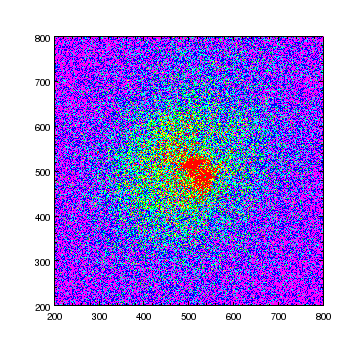### Figure 13: Using the logical coordinate system

The axes display the logical coordinate of the pixels (displayed as the Image coordinates in ds9). The user-specified limits (X is 200 to 800 and Y is 300 to 700) are adjusted to maintain the aspect ratio of the data, resulting in the range 200 to 800 along both axes (the Aspect ratios section below provides more examples).

Now we try the physical coordinate system which, as the dmlist output above, is called sky:

```chips> erase()
chips> add_image(f, ["depth", 50, "wcs", "sky"])
chips> set_cascading_property(chips_window, "color", "black")
chips> set_cascading_property(chips_window, "bgcolor", "white")
chips> set_image(["invert_colormap", True, "threshold", [0,15]])
chips> add_label(3800, 4600, "Abell 4059", ["color", "darkred"])
```

which creates Figure 14. We use the set_cascading_property calls to change all the color attributes in the window to black and the bgcolor attributes to white. This makes the on-screen version match the hardcopy output and can be useful if you want to see how the chosen color scheme or color map looks when printed out.

### Figure 14: Using the SKY coordinate system### Figure 14: Using the SKY coordinate system

Rather than use Ra and Dec for the axes, we now use X and Y. The label is added to the top-left of the plot since it uses this coordinate system.

As the color and bgcolor attributes have been swapped, the on-screen version looks the same as the hardcopy output (e.g. the PNG version).

### Aspect ratios

As previously discussed, images are created with their data aspect ratio set, as the output below shows

```chips> get_data_aspect_ratio()
'1:1'
chips> get_plot_aspect_ratio()
''
chips> get_plot_xrange()
[3557.14, 4582.139999999999]
chips> get_plot_yrange()
[3734.09, 4759.09]
```

Having the data aspect ratio set means that changes to either the axis limits or plot size will automatically change the display range to enforce the ratio. This is seen below where we use the split command to add an extra plot into the frame; since the plot area has changed (the width is the same but the height is now roughly half what it was) the data displayed in the plot has changed as shown by the change in plot ranges.

```chips> split(2, 1, 0.07)
chips> set_cascading_property(chips_plot, "color", "black")
chips> print(info_current())
Window [win1]
Frame [frm4]
Plot [plot2]
Coord Sys [Plot Normalized]

chips> get_plot_xrange("plot1")
[2939.1252518375322, 5200.1547481624675]
chips> get_plot_yrange("plot1")
[3734.09, 4759.09]
```

(the plot name is used in these calls since the split call changes plot currency to the last plot it displays, as shown in the info_current output). The Y axis has remained the same and the X axis has increased to compensate. The result is Figure 15.

### Figure 15: Changing the axis limits by calling split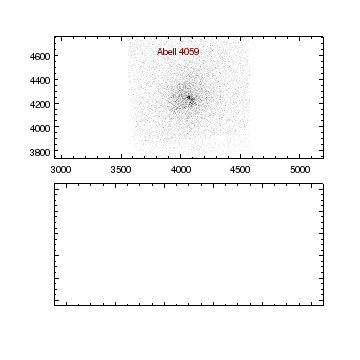### Figure 15: Changing the axis limits by calling split

Since the third argument to split was not zero there is a vertical gap between the two plots. Since the top plot is now rectangular, the X axis has been increased automatically to ensure the data aspect ratio constraint is maintained. We again use set_cascading_property to change the color attributes of the new plot (in particular the borders) to ensure they are visible, since they are created with color set to default. The actual color for default can be found from the preference settings:

```chips> get_preference("foreground.display")
'white'
```

We now change the plot aspect ratio to be square; this changes the plot borders and the axis limits (to keep the data aspect ratio constraint). The result of the following commands is shown in Figure 16.

```chips> set_plot_aspect_ratio("plot1", "1:1")
chips> get_plot_xrange()
[2939.1252518375322, 5200.1547481624675]
chips> get_plot_yrange()
[3116.0752518375325, 5377.1047481624682]
```

### Figure 16: Changing the plot aspect ratio### Figure 16: Changing the plot aspect ratio

The top plot has changed back to square, since it has both a '1:1' plot and data aspect ratio. The axis limits have again changed as the plot changed shape, although this time it is the Y axis that has been increased.

Since the limits for the first plot have changed significantly we use the limits command to get back to displaying the area covered by the image data:

```chips> limits(chips_image, "image1")
chips> split(1, 2, 0, 0.1)
```

The split call re-arranges the plots so they are now in a single row rather than column, as shown in Figure 17.

### Figure 17: Switching to horizontally-aligned plots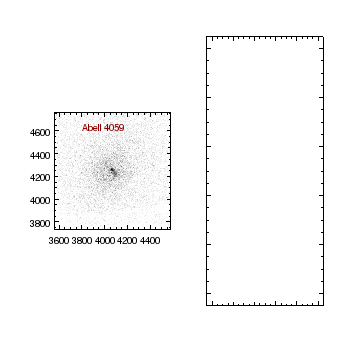### Figure 17: Switching to horizontally-aligned plots

The split call has re-arranged the plots (the fourth argument determines the spacing between columns and so governs the horizontal space between the two plots).

In the following we add the image to the second plot (as this also creates a pair of axes we need to change their color attributes to black to ensure they are visible on screen) and then remove the data aspect ratio from this plot.

```chips> add_image(f, ["depth", 50, "wcs", "sky"])
chips> set_image(["invert_colormap", True, "threshold", [0,15]])
chips> set_cascading_property(chips_axis, "color", "black")
chips> set_data_aspect_ratio('')
```

After removing the aspect ratio we change the plot currency to all and change the X axis of both plots to the range 3800 to 4200.

```chips> current_plot("all")
chips> limits(X_AXIS, 3800, 4200)
```

The result is shown in Figure 18.

### Figure 18: Removing the data aspect ratio from a plot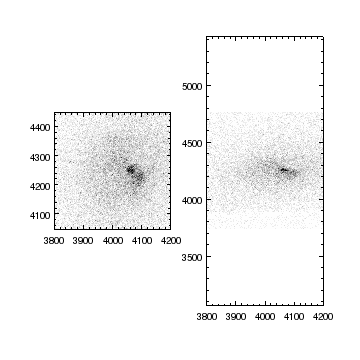### Figure 18: Removing the data aspect ratio from a plot

The left-hand plot has zoomed into the image since the reduction in range of the X axis requires the Y axis to be reduced too, to retain the data aspect ratio. Since the right-hand plot has no data aspect ratio, the Y axis is not affected by the limits call, and so the result is an image that appears stretched in the X direction.

We can illustrate the difference in the Y ranges of the two plots using the get_plot_yrange routine. If called with no argument you will get the following error, since multiple Y axes are current:

```chips> get_plot_yrange()
chips ERROR: 'All' is unsupported in get operations when more than one object exists
```

Explicit selection of the plots - in this case by providing the name as as argument - results in:

```chips> get_plot_yrange("plot1")
[4046.59, 4446.59]
chips> get_plot_yrange("plot2")
[3063.8976959227675, 5429.282304077233]
```

## Multiple images

In this section we create multiple plots, containing images (that happen to be the same data but could be different ones).

We start off by removing any existing ChIPS visualization and making a square window:

```chips> clear()
chips> add_window(8, 8, "inches")
```

We now use the read_file routine from the Crates moduel to load in the image and add it to the four plots created by the split call (see the creating and using multiple plots thread for more information on split):

```chips> cr = read_file("a4059_chandra.fits")
chips> split(2, 2)
chips> current_plot("all")
chips> add_image(cr)
```

The output of these commands is shown in Figure 19.

### Figure 19: Four images arranged in a 2 by 2 grid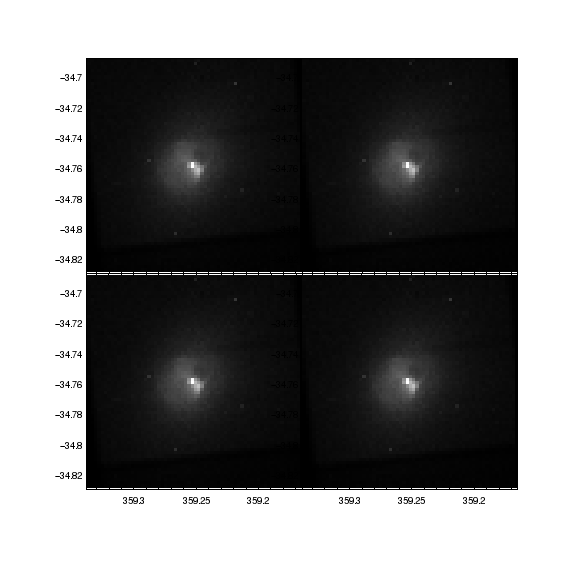### Figure 19: Four images arranged in a 2 by 2 grid

Since the four plots were created with no horizontal or vertical gaps between them, the numeric labels of the plots in the right column overlap the images in the left column. As with the very-first figure (Figure 1), the image within each plot overlaps the axes, causing some of the axes to be hidden.

Since the plot currency was set to "all" at the time of the add_image() call, the same image is added to each plot. As will be shown in later plots these are distinct copies, since you can change the attributes of a single image.

The info_current command can be used to determine what objects are current. The output here is:

```chips> print(info_current())
Window [win1]
Frame [frm1]
Plot [plot1]
Image [image1]
X Axis [ax1]
Y Axis [ay1]
Plot [plot2]
Image [image1]
X Axis [ax1]
Y Axis [ay1]
Plot [plot3]
Image [image1]
X Axis [ax1]
Y Axis [ay1]
Plot [plot4]
Image [image1]
X Axis [ax1]
Y Axis [ay1]

```

Unlike the previous plots, here we decide to hide the axes rather than re-arrange the depth of the images, which results in Figure 20.

```chips> set_plot(["style", "open"])
chips> hide_axis()
```

### Figure 20: Hiding the axes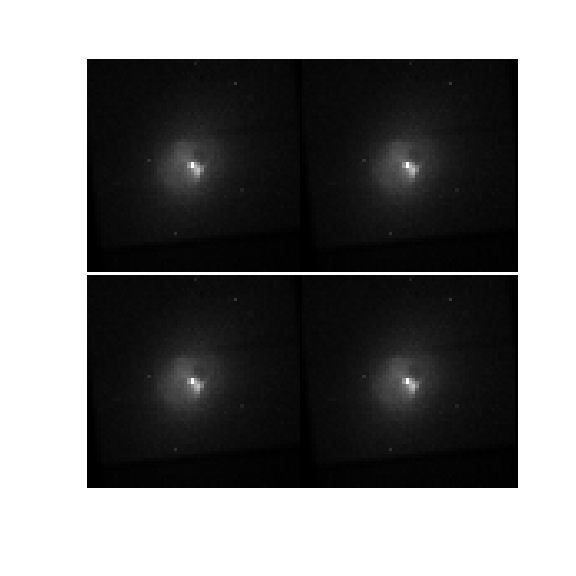### Figure 20: Hiding the axes

The set_plot call removes the borders from the display, leaving just the left Y axis and bottom X axis for each plot. These are then removed by the hide_axis() call.

We now decide to change the interpolation method used for displaying the image from its default (neighbor) to bilinear (this again is applied to all the images since the plot currency is still "all"):

```chips> set_image(["interpolation", "bilinear"])
```

An error is called if given an unsupported method:

```chips> set_image(["interpolation", "a"])
chips ERROR: The interpolation value (a) must be one of [neighbor, bilinear, bicubic]
```

The affect of this change can be seen by comparing Figure 20 and Figure 21.

### Figure 21: Changing the interpolation method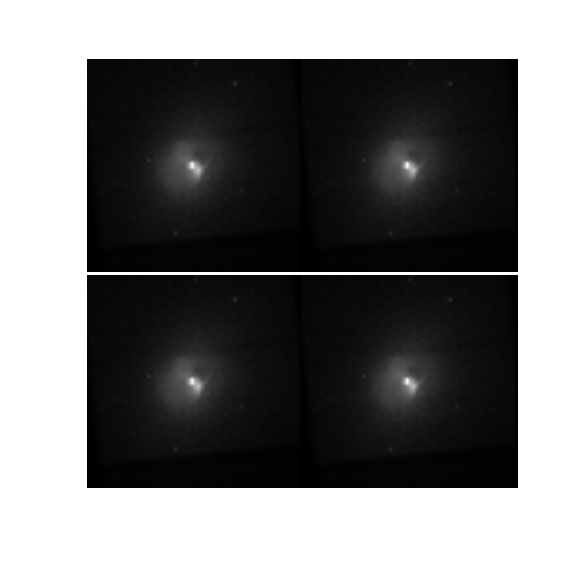### Figure 21: Changing the interpolation method

Changing from neighbor to bilinear produces a much smoother image than Figure 20.

We now want to annotate each plot with a label. Since we plan to use the same set up for each label we write a small Python routine called al:

```chips> def al(txt):
"Add a label at the top of the plot"
li = { "coordsys": PLOT_NORM, "size": 16,
"color": "orange", "halign": 0.5 }
add_label(0.5, 0.9, txt, li)

```

which can then be used as follows:

```chips> current_plot("plot1")
chips> al("grayscale")
chips> current_plot("plot2")
chips> set_image(["colormap", "heat"])
chips> al("heat")
chips> current_plot("plot3")
chips> set_image(["colormap", "cool"])
chips> al("cool")
chips> current_plot("plot4")
chips> set_image(["invert_colormap", "True"])
chips> al("inverted")
```

noting that the default plot labeling goes from left to right, then top to bottom (so "plot2" is the top-right plot).

We now want to change the scaling, so we again use dmstat, this time taking advantage of the runtool module for running CIAO tools from Python. First we need to make sure the relevant code is loaded:

```chips> from ciao_contrib.runtool import dmstat
```

If this step fails please make sure that you have the latest version of the CIAO scripts and modules installed.

The dmstat tool can be run using the routine; here we hide the screen output and get the minimum and maximum pixel values from the out_min and out_max fields of the object (this image is binned heavily, sixteen times the pixel scale used earlier, which is why the maximum pixel value is significantly larger):

```chips> dmstat("a4059_chandra.fits", centroid=False, verbose=0)
chips> dmstat.out_min
'0'
chips> dmstat.out_max
'2517'
```

As we want to apply the same scaling to each image, we change back to making all plots current before calling set_image:

```chips> current_plot("all")
chips> zoom(2)
chips> set_image(["threshold", [100, 2200]])
```

The result is Figure 22.

### Figure 22: Changing the color maps### Figure 22: Changing the color maps

The Multiple Color Maps example in the ChIPS gallery shows the list of available color maps. The Using Contrib Color Look-Up Tables thread shows how you can use the large number of look-up tables provided with the CIAO contrib package (see \$ASCDS_CONTRIB/data).

We now add color bars to each image, to show off support for vertical alignment:

```chips> opts = {"orientation": "vertical", "length": 0.5}
chips> current_plot("plot1")
chips> add_colorbar(-0.05, 0.75, opts)
chips> current_plot("plot3")
chips> add_colorbar(-0.05, 0.25, opts)
chips> current_plot("plot2")
chips> add_colorbar(1.05, 0.75, opts)
chips> current_plot("plot4")
chips> opts["*.color"] = "black"
chips> add_colorbar(0.8, 0.3, opts)
```

As Figure 23 shows, the color bars can be placed within a plot.

### Figure 23: Adding color bars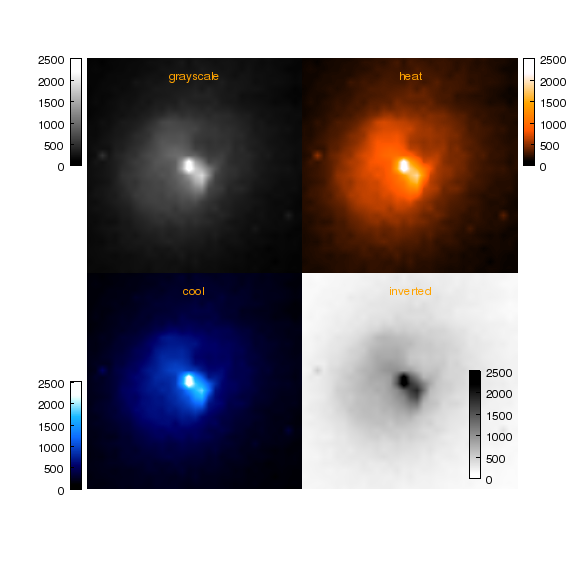### Figure 23: Adding color bars

Each image now has its own colorbar; the add_colorbar call uses the current image to determine what colormap to display (and the range) in the color bar.

For the bottom-right plot, where we place the colorbar over the image, we change all the color attributes from default to black to ensure the object is visible.

The color bars have a number of attributes, as shown below:

```chips> get_colorbar()

border.visible = True
depth = 100
halign = 0.5
id = None
label.angle = 270.0
label.color = black
label.font = helvetica
label.fontstyle = normal
label.halign = -99.0
label.location = inside
label.size = 12
label.text = None
label.valign = -99.0
label.visible = True
length = 0.5
offset.parallel = 0.0
offset.perpendicular = 5.0
orientation = 1
stem = None
tick.color = black
tick.length = 4
tick.location = outside
tick.mode = nice
tick.style = inside
tick.thickness = 1.0
tick.visible = True
ticklabel.angle = 0.0
ticklabel.color = black
ticklabel.font = helvetica
ticklabel.fontstyle = normal
ticklabel.halign = -99.0
ticklabel.offset = 6
ticklabel.size = 12
ticklabel.valign = -99.0
ticklabel.visible = True
valign = 0.5
width = 0.0500000007451

```

## Modifying image data

Most of the images above were created by giving a file name to make_figure or add_image, but you can also display a Crate (e.g. Figure 19) or a NumPy array. In the following section we show several examples of this support, including modifying pixel values (e.g. smoothing) before display.

We start by loading in the utils module for Crates, provided as part of the CIAO scripts and modules package.

```chips> from crates_contrib.utils import *
```

We now load in the image data using a Crates routine; the return value is an object which can be used to get image data, metadata or as an argument in add_image calls.

```chips> cr = read_file('a4059_chandra_bin1.fits')
chips> cr

Crate Type:        <IMAGECrate>
Crate Name:        IMAGE
```

Before we display the image, we decide to smooth it with a gaussian, using the smooth_image_crate routine:

```chips> smooth_image_crate(cr, "gauss", 5)
chips> add_image(cr, ["depth", 50, "colormap", "hsv"])
chips> add_colorbar(1.05, 0.5, ["orientation", "vertical"])
chips> print(info())
Window [win1]
Frame [frm1]
Plot [plot1]   (0.15,0.15)  .. (0.90,0.90)
Border bottom [bx1]  top [bx2]  left [by1]  right [by2]
Image [image1]
X Axis [ax1]
Y Axis [ay1]
ColorBar [cbar1]

chips> set_plot_aspect_ratio("1:1")
chips> set_plot(["rightmargin", 0.15])
```

The result is shown in Figure 24 (the plot aspect ratio was set so that the plot would remain square when the right margin was increased).

### Figure 24: Smoothing an image### Figure 24: Smoothing an image

In the following we have decided that we do not want to display negative values, and so use NumPy commands to change any pixel with a value less than 0 to 0:

```chips> pvals = get_piximgvals(cr)
chips> pvals.min()
-5.4887734982078662e-16
chips> pvals.max()
12.083261451204574
chips> pvals[pvals < 0] = 0
chips> pvals.min()
0.0
```

The get_piximgvals call returns a NumPy array of the data stored in the Crate. Since we did not use copy_piximgvals, this is the actual data stored in the Crate, so any changes made to the pixel values are also made to the Crate; we take advantage of this fact to change all the negative pixel values to 0 using the syntax pvals[pvals < 0] = 0. The result on the data stored in the crate can be seen with:

```chips> get_piximgvals(cr).min()
0.0
```Note

An alternative would have been to copy the pixel values, edit this copy, and then store the copied values back in the crate using:

```chips> pvals = copy_piximgvals(cr)
chips> pvals[pvals < 0] = 0
chips> set_piximgvals(cr, pvals)
1
```

In the following we re-display the Crate (and hence use the adjusted pixel values). We also make several other changes, including moving the axes slightly away from the data (this is an alternative to the techniques used earlier (Figure 4) to ensure the tick marks remain visible):

```chips> erase()
chips> add_image(cr, ["depth", 50, "colormap", "hsv"])
chips> add_colorbar(1.05, 0.5, ["orientation", "vertical"])
chips> set_plot_aspect_ratio("1:1")
chips> set_plot(["rightmargin", 0.15])
chips> set_cascading_property(chips_plot, "color", "brown")
chips> move_axis(-0.05, -0.05)
chips> set_plot(["style", "open"])
chips> set_axis(["tickformat", "%.2f"])
```

The final version is shown in Figure 25.

### Figure 25: Adjusting pixel values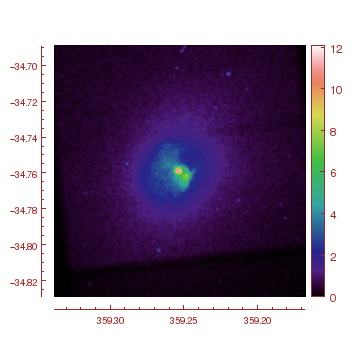### Figure 25: Adjusting pixel values

The X and Y axes have been moved away from image data by the move_axis calls. The axes were created using the plot-normalized coordinate system, so that is the system used to interpret the coordinates given to move_axis; here we move the X axis to just below the plot (yPLOT_NORM=-0.05) and the Y axis to the left (xPLOT_NORM=-0.05).

Taking advantage of the ChIPS GUI, the axes can also be moved by selecting them and then either dragging them with the mouse or using the arrow keys.

The reason for using the Crate as the argument to the add_image call is that this provides useful extra information read in from the file, in particular the WCS transformation needed to create axes of Right Ascension and Declination. An alternative is to use the pixel value array could have been used directly, as shown in Figure 26:

```chips> erase()
chips> opts = { "depth": 50, "colormap": "hsv" }
chips> add_image(np.arcsinh(pvals), opts)
chips> add_colorbar(0.5, 1.05)
chips> set_image(["threshold", [0.2, 3]])
```

### Figure 26: Displaying a NumPy Array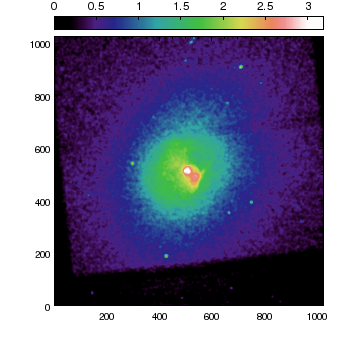### Figure 26: Displaying a NumPy Array

Since ChIPS only supports a linear scale for image visualizations, we use the NumPy routines to modify the pixel values before displaying them; in this case we display the inverse hyperbolic sine values which highlights the low-level structure in the data.

Unlike the Crates examples earlier - e.g. Figure 25 - the image is displayed in logical coordinates.

Note that the color bar and threshold values use the displayed pixel range (so here 0 to just over 3 rather than the 0 to 12 of pvals).

Note that the scale_image_crate routine can be used to scale the pixel values stored in an image crate, just as smooth_image_crate is used to smooth the values.

In order to display the correct WCS coordinates, we use the get_transform command to extract the transformation from the crate:

```chips> sky = get_transform(cr, 'SKY')
chips> print(sky.print_parameter_list())
Name: SCALE	Type: dmDOUBLE	Value: 1.000000, 1.000000	   	Desc: Scale	Parent: sky
Name: ROTATION	Type: dmDOUBLE	Value: 0.000000	   	Desc: Rotation Angle	Parent: sky
Name: OFFSET	Type: dmDOUBLE	Value: 3556.640000, 3734.090000	   	Desc: Origin Offset	Parent: sky
```

which can then be used in the add_image call, to create Figure 27:

```chips> erase()
chips> opts['threshold'] = [0.2, 3]
chips> add_image(np.arcsinh(pvals), opts)
```

### Figure 27: Displaying a NumPy Array: adding a sky coordinate system### Figure 27: Displaying a NumPy Array: adding a sky coordinate system

This is the same figure as Figure 26 apart from the fact that the axis limits are now around 4000 rather than 500 (and the color bar has not been added).

```chips> print(get_plot_xrange())
[3557.14, 4582.139999999999]
chips> print(get_plot_yrange())
[3734.09, 4759.09]
```

The same approach can be used to use the WCS coordinate system:

```chips> eqpos = get_transform(cr, 'EQPOS')
chips> print(eqpos.print_parameter_list())
Name: CRPIX	Type: dmDOUBLE	Value: 4096.500000, 4096.500000	   	Desc: Reference Point Pixel Coordinates	Parent: EQPOS
Name: CRVAL	Type: dmDOUBLE	Value: 359.248199, -34.779542	   	Desc: Center Coordinate in decimal degrees	Parent: EQPOS
Name: CDELT	Type: dmDOUBLE	Value: -0.000137, 0.000137	   	Desc: Transform Scale in degrees/pixel	Parent: EQPOS
Name: CROTA	Type: dmDOUBLE	Value: 0.000000	   	Desc: Rotation Angle	Parent: EQPOS
Name: EQUINOX	Type: dmSHORT	Value: 2000	   	Desc: Equinox of Coordinates	Parent: EQPOS
Name: EPOCH	Type: dmDOUBLE	Value: 2000.000000	   	Desc: Epoch of Coordinates	Parent: EQPOS
Name: CTYPE	Type: dmCHAR	Value: RA---TAN   , DEC--TAN	   	Desc: Coordinate Projection Type	Parent: EQPOS
```

and we now decide to use a logarithmic scaling for the image (the warning message will not appear if you have already seen it in the ChIPS session, and can be controlled with the np.seterr routine):

```chips> lvals = np.log10(pvals)
/soft/ciao-4.8/ots/bin/ipython:1: RuntimeWarning: divide by zero encountered in log10
#!/usr/bin/env python
```
```chips> lvals.min()
-inf
chips> lvals.max()
1.08218417262
chips> lvals[lvals > -np.inf].min()
-17.436615949663771
```

While ChIPS will ignore NaN values it does not handle the np.inf and -np.inf as well, so we ignore these (and all other pixels with a small value) by saying

```chips> lvals[lvals < -2] = -2
```

and the data plotted with the following, which also moves the plot, adds a colorbar below the plot, and changes the colormap to use the cubehelix color scheme, to create Figure 28:

```chips> erase()
chips> opts['threshold'] = [-2, 1]
chips> add_image(lvals, eqpos, opts)
chips> set_xaxis(['tickformat', 'ra1'])
chips> set_yaxis(['tickformat', 'dec1'])
chips> move_plot(0.05, 0.05, 1)
chips> import chips_contrib.helix as helix
chips> helix.load_colormap_cubehelix()
chips> set_image(['colormap', 'usercmap1'])
chips> set_preferences(['foreground.display', 'black', 'background.display', 'white'])
chips> move_axis(-0.05, -0.05)
```

### Figure 28: Displaying a NumPy Array: adding a tangent-plane coordinate system### Figure 28: Displaying a NumPy Array: adding a tangent-plane coordinate system

Since the 'EQPOS' transform was used, the axes are in Right Ascension and Declination, using the tangent-plane projection (this is not obvious here - other than the fact that the X axis is in descending order - since the field is not near a pole so that the distortions are not large; zooming out a large distance will make this clearer).

The following section describes the reason behind changing the foreground.display and background.display preference settings.

The red, green, and blue components of the colormap can be retrieved with the helix.get_cubehelix routine, and passed to load_colormap. In the following we also add in an array to represent the alpha value of each color; in this case all but the first are fully opaque (alpha=1), while the first value is fully transparent (alpha=0). The result is that the really-low values (i.e. those who were reset to a value of -2 above), are no longer displayed (Figure 29):

```chips> (r, g, b) = helix.get_cubehelix()
chips> alpha = np.ones(r.size)
chips> alpha = 0
chips> load_colormap(r, g, b, alpha, chips_usercmap2)
chips> set_image(['colormap', 'usercmap2'])
chips> set_plot(['style', 'open'])
```

### Figure 29: Displaying a NumPy Array: using opacity to remove unwanted pixel values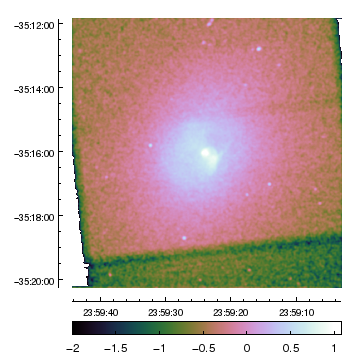### Figure 29: Displaying a NumPy Array: using opacity to remove unwanted pixel values

Note that transparency is not supported in the postscript or EPS outputs.

If a colormap - one of chips_usercmap1, chips_usercmap2, or chips_usercmap3 is changed by a call to load_colormap then any images using that colormap will need to have their colormap attribute re-set for this change to affect existing images.

## Combining images and annotations

In this section we will display contours of the Chandra data of Abell 4059 on top of a GALEX image of the cluster, coupled with a number of annotations. It provides an example of using the smooth_image_crate routine and converting between different display coordinate systems using the convert_coordinate command.

Before starting on the visualization we change the ChIPS preferences so that the on-screen display matches that used in the hardcopy outputs. This is an alternative to the technique used above (Figure 14).

```chips> clear()
chips> set_preference("foreground.display", "black")
chips> set_preference("background.display", "white")
chips> add_window(8,8,"inches")
```

We now read in the GALEX data, smooth it slightly, and display it (the threshold only covers a small range of the data since the BCG is faint here). We also adjust the color map (including inverting it), the axes, add gray grid lines and zoom into the image slightly.

```chips> galex = read_file("a4059_galex.fits")
chips> smooth_image_crate(galex, "gauss", 3)
chips> add_image(galex, ["depth", 50, "threshold", [0,0.02]])
chips> set_image(["colormap", "heat"])
chips> set_image(["invert_colormap", True])
chips> set_xaxis(["tickformat", "ra"])
chips> set_yaxis(["tickformat", "dec"])
chips> set_axis(["majorgrid.visible", True, "majorgrid.color", "gray"])
chips> zoom(1.2)
```

Since the GALEX image is large, we decide to overlay the full field-of-view of the Chandra observation (observation id: 5785), where a4059_fov1.fits is the renamed fov1 file from the Chandra archive:

```chips> from chips_contrib.regions import *
chips> add_fov_region("a4059_fov1.fits")
chips> set_region("all", ["opacity", 0.2])
```

which creates Figure 30.

### Figure 30: Overlaying a region on an image### Figure 30: Overlaying a region on an image

The on-screen, PNG, and PDF version of the visualization support transparency, so the green regions indicating the Chandra observation show the underlying GALEX data as well. The PS output does not support transparency, and so in this version the green regions are displayed with a solid fill.

We turn off the region fill and make the edges thicker, so that they are more visible with the following command:

```chips> set_region(["fill.style", "nofill", "edge.thickness", 2])
```

Since the GALEX image is so large, we now add a zoomed-in version of the data as a "picture in picture", by first creating a new plot and then displaying the image in it (although we use the same threshold and color map as above we decide not to invert this version). The zoom factor was chosen to dislplay just the central region covered by the Chandra data. Later on we shall add a region and lines to the main plot to visually link these plots.

```chips> add_plot(0.6, 0.2, 0.85, 0.45)
chips> set_plot(["style", "open"])
chips> add_image(galex, ["threshold", [0,0.02]])
chips> set_image(["colormap", "heat"])
chips> hide_axis()
chips> zoom(10)
```

We now add in contours of the X-ray emission:

```chips> add_contour("a4059_chandra.fits", ["color", "white"])
chips> set_contour(["levels", [500, 750, 1000, 2000]])
```

We want to finish by adding an outline to the main image indicating the area covered by the inset plot. To do this we need the coordinates of the corners of the second plot, which we can get using

```chips> xr = get_plot_xrange()
chips> yr = get_plot_yrange()
chips> xbox = [xr, xr, xr, xr]
chips> ybox = [yr, yr, yr, yr]
```

The xbox and ybox arrays give the coordinates of the four corners of the second plot in data coordinates. We also want to draw lines connecting two of these corners, so we use the convert_coordinate command to calculate the coordinates of the plot corners in the frame-normalized system using:

```chips> f1 = convert_coordinate([0,0], PLOT_NORM, FRAME_NORM)
chips> f2 = convert_coordinate([1,1], PLOT_NORM, FRAME_NORM)
chips> f1
[ 0.60000002  0.2       ]
chips> f2
[ 0.85000002  0.44999999]
```

So, f1 and f2 contain the coordinates of the bottom-left and top-right corners of the inset plot using the frame-normalized coordinate system. In this particular case we didn't actually need to do this calculation since the coordinates used in the add_plot call above are in the desired coordinate system!

As a reminder, the ChIPS objects that exist in the visualization are:

```chips> print(info())
Window [win1]
Frame [frm1]
Plot [plot1]   (0.15,0.15)  .. (0.90,0.90)
Border bottom [bx1]  top [bx2]  left [by1]  right [by2]
Image [image1]
X Axis [ax1]
Y Axis [ay1]
Region [obsid5785-ccd2]
Region [obsid5785-ccd3]
Region [obsid5785-ccd5]
Region [obsid5785-ccd6]
Region [obsid5785-ccd7]
Plot [plot2]   (0.60,0.20)  .. (0.85,0.45)
Border bottom [bx1]  top [bx2]  left [by1]  right [by2]
Image [image1]
X Axis [ax1]
Y Axis [ay1]
Contour [ctr1]

chips> print(info_current())
Window [win1]
Frame [frm1]
Plot [plot2]
Image [image1]
X Axis [ax1]
Y Axis [ay1]
Contour [ctr1]
Coord Sys [Data]
Coord Sys ID [ds3.8.13.55]

```

We now move back to the first image (which is in the first plot) and add a region to indicate the area covered by the inset plot:

```chips> current_plot("plot1")
chips> add_region(xbox, ybox, ["fill.style", "nofill"])
chips> set_region(["edge.thickness", 2, "edge.color", "black"])
```

We finish off by drawing lines between the top-right and bottom-left corners of the region and the inset plot. We could position these lines using the frame-normalized coordinate system, but we instead chose to use the plot-normalized system, which requires converting the f1 and f2 values calculated above -

```chips> s1 = convert_coordinate(f1, FRAME_NORM, PLOT_NORM)
chips> s2 = convert_coordinate(f2, FRAME_NORM, PLOT_NORM)
chips> s1
[ 0.60000005  0.06666667]
chips> s2
[ 0.93333339  0.39999999]
```

- and then finding out the plot-normalized coordinates of the region we just added:

```chips> e1 = convert_coordinate([xr,yr], DATA, PLOT_NORM)
chips> e2 = convert_coordinate([xr,yr], DATA, PLOT_NORM)
```

We can now add lines connecting s1 and e1 and between s2 and e2 using the following to create Figure 31:

```chips> opts = ["coordsys", PLOT_NORM, "style", "shortdash"]
chips> add_line(s1, s1, e1, e1, opts)
chips> add_line(s2, s2, e2, e2, opts)
chips> set_plot_title("plot1", "Chandra contours on GALEX image")
```

### Figure 31: Adding a zoomed-in version of the image### Figure 31: Adding a zoomed-in version of the image

The dotted lines connecting the two plots are only correct for the current plot limits; if the limits are changed - e.g. by zoom, limits, or via dragging within the plot - then the lines will need to be deleted and new coordinates calculated.

## History

 15 Dec 2010 New for CIAO 4.3 15 Dec 2011 Updated for CIAO 4.4: added an example of the new ChIPS GUI (Figure 3 and Figure 8); noted the support for opacity and alpha settings in the PDF output (Figure 7 and Figure 30). 13 Dec 2012 Updated for CIAO 4.5: added a link to the Using Contrib Color Loop-Up Tables thread in the caption to Figure 22. 05 Dec 2013 Updated for CIAO 4.6: noted the change in how non tangent-plane projection systems are handled; noted the change in behavior with axis labels and the new x.label and y.label attributes for axes; noted the change in behavior of the foreground.display and background.display background settings; added examples showing how to add coordinate transforms to a plot (Figure 27 and Figure 28) and how to use transparency to hide areas of an image (Figure 29). 10 Dec 2014 Updated for CIAO 4.7. 15 Dec 2015 Updated for CIAO 4.8.